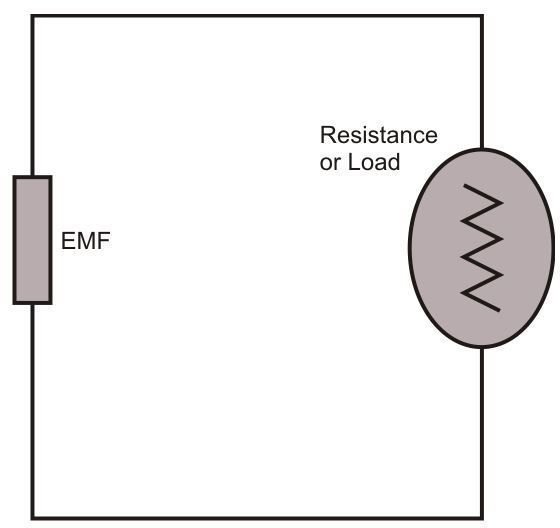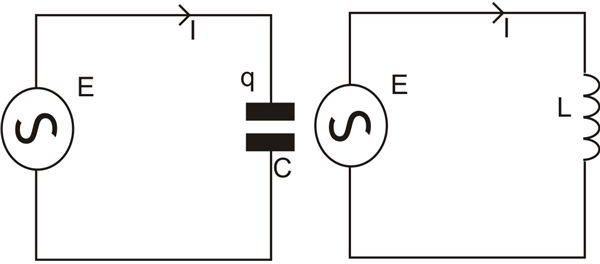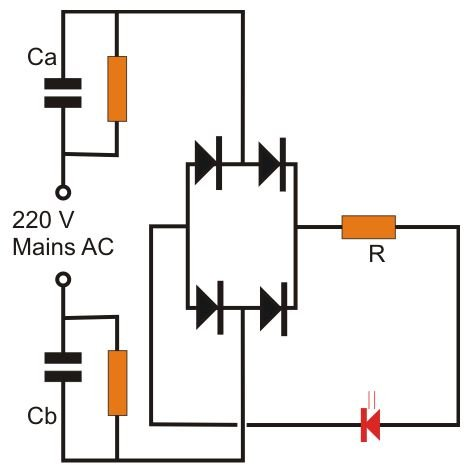# How to Calculate the Values of Current, Voltage, and Resistance in a Circuit?

I have discussed a number of interesting electronic circuits and projects in Bright Hub – some being very simple and others not so simple. Though I’ve tried my best to answer and solve the problems and curiosity shown by readers, with the electronic subject being so vast, the explanations provided so far keep looking incomplete.

In the course of this ongoing effort I’ve tried to produce yet another article- this one!- dedicated to one of reader’s Patrick Vaz request as to how would one can calculate the values of current, voltage, and resistance in a circuit without employing difficult to understand and complex formulas, or simply, through easy, straightforward expressions.

Let's begin the discussion by refering to the figure below. It shows the most basic form of an electrical circuit, consisting a of bulb and a battery (electromotive force). Calculating the potential drop (voltage across the load), current, and the resistance is very simple here, as we all know, it can be done using Ohm’s law:

Er = RI,

Where Er is the voltage drop across the load, a bulb here (resistive) and is proportional to I, the instantaneous current and R is the constant of proportionality, more popularly known as resistance.

If we suppose the applied voltage here to be 3 volts and the current consumed by the bulb to be 200mA, then the resistance using the above formula can be calculated as: 3 = R × 0.2, therefore R = 15 Ω – pretty elementary isn't that?Circuits involving inductors and capacitors are more complex in their behavior. Typically an inductor will always oppose a change in current passing through it, in a mechanical analogy it may be quite compared to inertia generated in a moving mass. Referring to the next circuit which includes an inductor, according to Ohm’s law the voltage drop aross the inductor is proportional to the rate of change of current with respect to time.

Therefore, Eɩ = LdI/dt, where L is the constant of proportionality. It is called inductance and is measured in henrys.

For a capacitor the voltage drop is proportional to the instantaneous electric charge on the capacitor, i.e. Ec = Q/C, where C is the capacitance and Q is the charge. C is measured in farads and Q in coulombs

## What is Reactance in Inductors and Capacitors?

As discussed in the previous section, an inductor always opposes and tries to balance a change in current. Analyzing this factor through the diagram shown below, we find that the inductor L when subjected to an AC balances a rise in the magnitude of current by an inducing an opposite opposing potential (negative voltage) and when the current value drops, the inductor tries to pull it to the initial magnitude by supplying a positive potential with the flow of the current. The plotted graph simply illustrates the voltage and current pattern across the inductor. This tendency of an inductor is called its reactance and is given as:

Xɩ = 2πfL

Where f is the voltage frequency in Hz, L is the inductance in mill henrys, and is in Ohms. So basically for inductors and capacitors the reactance value is equivalent to the “resistance” produced in the path of the applied AC potential. Once we calculate the reactance, the current in the circuit can be simply calculated by using Ohm’s law.

For inductors the reactance is directly proportional to the induced frequency.

However for capacitors the reactance is given as:

Xɩ = 1/2πfC, which clearly shows that it’s inversely proportional to the induced frequency, means that as the frequency increases the reactance decreases and vice versa.We can apply the above formulas for calculating voltage, current, resistance (reactance for capacitors and inductors) in the following example; this will help us to understand the applications for many other different configurations universally.

The example circuit diagram shown below is an innovative capacitive power supply design; the two capacitors at the two inputs ensure that the power supply remains aloof from the dangerous AC potentials and safe from electric shocks (designed by me).Here we may be interested first to find out the current supplying capacity of the circuit. For this it becomes important to find the resistance or the impedance offered by the capacitors to the applied AC. Since the capacitors are in series the net capacitance can be calculated as:

Assuming Ca and Cb both = 1µ/400V

1/C = 1/Ca + 1/Cb

= 1/1 + 1/1 = 2

Therefore net Capacitance = 0.5µF or 0.0000005F

Using the reactance formula for capacotors:

Xɩ = 1/2πfC = ½ × 3.14 × 50 × (0.0000005) = 6369.4 Ohms,

Now using Ohm’s law:

Er = RI,

220 = 6369.4I,

Therefore I = 35mA,

The above result provides the information regarding the maximum current output of the power supply @ 220V, after rectification the output becomes 220V DC, 35mA. The voltage though may look huge, practically its found that due to maximum current restricted @ 35mA, the voltage drops drastically when a load is connected here. However since for an LED even this output may be potentially harmful, an addition of a resistor becomes imperative. The resistance value may be calculated by using the following formula:

R = (supply voltage VS – LED forward voltage VF) / LED current IL,

Therefore, R = (220 – 3.5)/0.020 = 10825 Ω or 10 K, however practically you will find that any value above 100 Ohms works adequately well due to the low current involved with the circuit. If you are using more LEDs in series, the forward voltage just needs to be modified accordingly, the remaining parameters can be kept as it is. For example if six LEDs are used the forward voltage drop across them becomes = 3.5 × 6 = 21 volts, which can be used in the above formula for calculating the value of the series resistor.